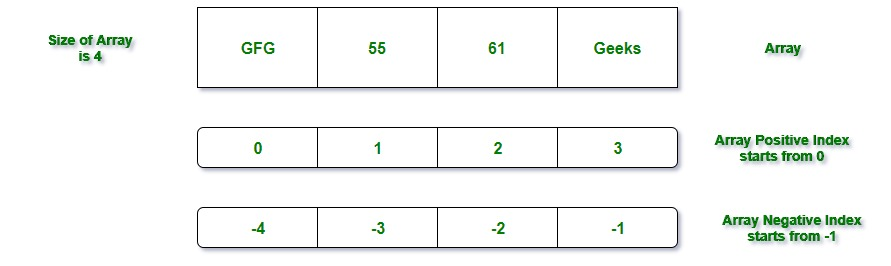# Ruby | Arrays

An array is a collection of different or similar items, stored at contiguous memory locations. The idea is to store multiple items of the same type together which can be referred to by a common name.
In Ruby, numbers, strings, etc all are primitive types but arrays are of objects type i.e arrays are the collection of ordered, integer-indexed objects which can be store number, integer, string, hash, symbol, objects or even any other array. In general, an array is created by listing the elements which will be separated by commas and enclosed between the square brackets[].

Example:

`["Geeks", 55, 61, "GFG"]`Here array contains the 4 elements of different type i.e Geeks(a string), 55(number), 61(number), and GFG(string). Array positive index starts from 0. The negative index always starts with -1 which represents the elements from the end of the array. There can be 2-Dimensonal array i.e array will store another array. It is also termed as the nested arrays. Here we will only discuss about 1-Dimensional arrays.

Creation of 1-D array in Ruby

There are several ways to create an array. But there are two ways which mostly used are as follows:

1. Using the new class method: new is a method which can be used to create the arrays with the help of dot operator. Here ::new method with zero, one or more than one arguments is called internally. Passing arguments to method means to provide the size to array and elements to array.

Syntax:

`name_of_array= Array.new`

Example:

`arr = Array.new`

Here arr is the name of the array. Here Array is the class name which is predefined in the Ruby library and new is the predefined method.

Note: To find the size or length of an array just use either size method or length method . Both methods will return same value for a particular array.

```arr = Array.new(40)
arr.size
arr.length
```

Now array can hold 40 elements. Here 40 is the size & the length of the array.

Program:

 `# Ruby program to demonstrate the  ` `# creation of array using new method ` `# and to find the size and length of array ` ` `  `# creating array using new method  ` `# without passing any parameter ` `arr = ``Array``.``new``() ` ` `  `# creating array using new method  ` `# passing one parameter i.e. the  ` `# size of array ` `arr2 = ``Array``.``new``(``7``) ` ` `  `# creating array using new method  ` `# passing two parameters i.e. the  ` `# size of array & element of array ` `arr3 = ``Array``.``new``(``4``, ``"GFG"``) ` ` `  `# displaying the size of arrays ` `# using size and length method ` `puts arr.size ` `puts arr2.length ` `puts arr3.size ` ` `  `# displaying array elements ` `puts ``"#{arr3}"`

Output:

```0
7
4
["GFG", "GFG", "GFG", "GFG"]
```
2. Using literal constructor[] In Ruby, [] is known as the literal constructor which can be used to create the arrays. Between [], different or similar type values can be assigned to an array
Example:

 `# Ruby program to demonstrate the  ` `# creation of array using literal  ` `# constructor[] and to find the size  ` `# and length of array ` ` `  `# creating array of characters ` `arr = ``Array``[``'a'``, ``'b'``, ``'c'``, ``'d'``,``'e'``, ``'f'``] ` ` `  `# displaying array elements ` `puts ``"#{arr}"` ` `  `# displaying array size ` `puts ``"Size of arr is: #{arr.size}"` ` `  `# displaying array length ` `puts ``"Length of arr is: #{arr.length}"`

Output:

```["a", "b", "c", "d", "e", "f"]
[1, 2, 3, 4, 5, 6, 7]
Size of arr is: 6
Length of arr is: 6
```

Retrieving Or Accessing Elements from Array

In Ruby, there are several ways to retrieve the elements from the array. Ruby arrays provide a lot of different methods to access the array element. But the most used way is to use the index of an array.

Example:

 `# Ruby program to demonstrate the  ` `# accessing the elements of the array ` ` `  `# creating string using [] ` `str = [``"GFG"``, ``"G4G"``, ``"Sudo"``, ``"Geeks"``] ` ` `  `# accessing array elements ` `# using index ` `puts str[``1``] ` ` `  `# using the negative index ` `puts str[-``1``] `

Output:

```G4G
Geeks
```

Retrieving Multiple Elements from Array: There can be many situations where the user need to access the multiple elements from the array. So to access the multiple elements, pass the two specified array index into the [].

Example:

 `# Ruby program to demonstrate the  ` `# accessing the multiple elements  ` `# from  array ` ` `  `# creating string using [] ` `str = [``"GFG"``, ``"G4G"``, ``"Sudo"``, ``"Geeks"``] ` ` `  `# accessing multiple array elements ` `puts str[``2``,``3``] `

Output:

```Sudo
Geeks
```

Note: If the user will try to access the element that doesn’t exist in the array then nil will return.

Example:

 `# Ruby program to demonstrate the accessing  ` `# of array element that doesn't exist ` `  `  `# creating array using [] ` `arr = [``1``, ``2``, ``3``, ``4``] ` ` `  `# accessing the index which  ` `# doesn't exist ` `puts arr[``4``] `

There will be nothing in the output.

My Personal Notes arrow_drop_upCheck out this Author's contributed articles.

If you like GeeksforGeeks and would like to contribute, you can also write an article using contribute.geeksforgeeks.org or mail your article to contribute@geeksforgeeks.org. See your article appearing on the GeeksforGeeks main page and help other Geeks.

Please Improve this article if you find anything incorrect by clicking on the "Improve Article" button below.

Improved By : Akanksha_Rai

Article Tags :

1

Please write to us at contribute@geeksforgeeks.org to report any issue with the above content.